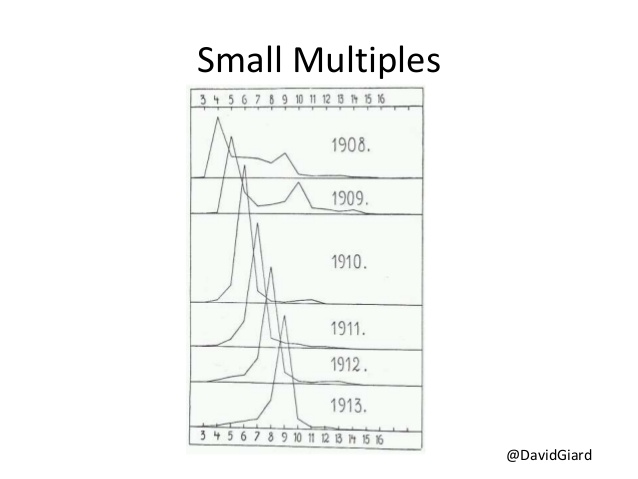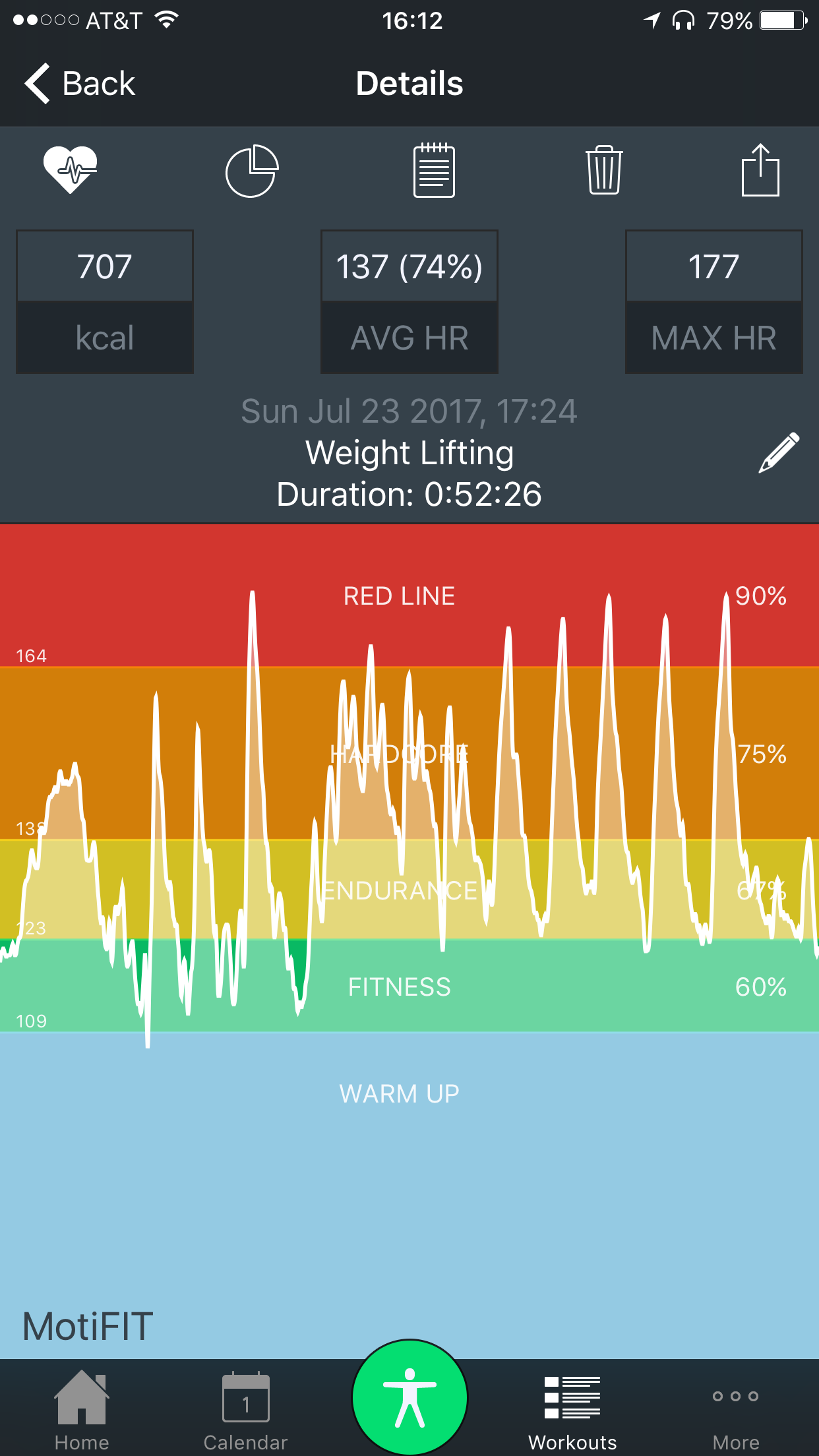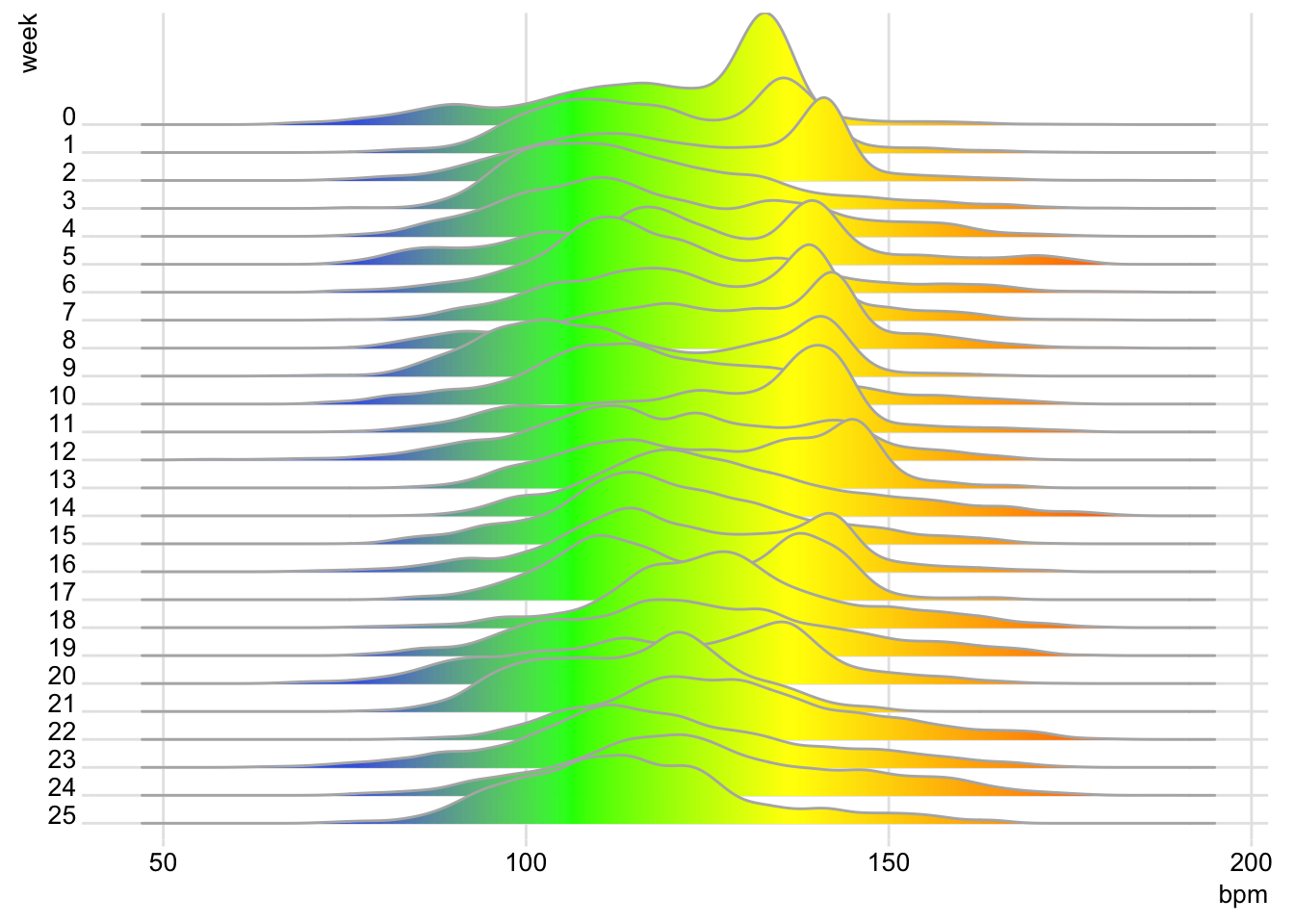# The “joy” of plotting heartrate dataThere’s been quite a few posts lately in the R world about the joyplot. I emailed my dataviz instructor from last fall this wonderful post that shows how Congress has become more polarized on one side of the aisle. He pointed out this isn’t as new as everyone thinks it is, as Tufte gives an example of it in one of his books from the early 1900s (this image is from slide 71 of this presentation).

In any event, joyplots are great for comparing values over time. I’ve been wearing a heartrate monitor while at the gym since February, and the MotiFit app lets you export that data to a CSV file. I’ve been saving these files each day after working out, but I haven’t done much with it yet. After gathering 6 months of data, it seemed high time to play around with joyplots!

The first thing I needed to do was combine all the files, which wasn’t as straightforward as I hoped. The MotiFit app exported data in two different formats and two different encodings, so I had to account for this. I wound up guessing the encodings from the readr package, and then combining the date and time columns where necessary.

``````out.file <- data.frame()
file.names <- dir(datadir, pattern = "HeartRateData.+?.csv") # You'll need to set datadir

for (i in 1:length(file.names)){
# exported files are in at least 2 different encodings, so we're going to guess using the guess_encoding function from the readr package
encoding <- guess_encoding(paste(datadir, "/", file.names[i], sep=""), n_max = 1000)
skip = 1,
stringsAsFactors = FALSE,
fileEncoding = toString(encoding[1,1])
)

# exported data has 2 or three columns, so if it's three, we're going to join the date and time fields
if (length(file) > 2) {
temp.date <- file[,1]
temp.time <- trimws(file[,2])
temp.bpm <- trimws(file[,3])

# date format is Wed Feb 8 2017
temp.datetime <- as.POSIXct(paste(temp.date, temp.time, sep=" "), format = "%a %b %d %Y %H:%M")

temp.df <- data.frame(temp.datetime, temp.bpm)
names(temp.df) <- c("timestamp", "bpm")
file <- temp.df
rm(temp.df)
} else {
names(file) <- c("timestamp", "bpm")
file\$timestamp <- as.POSIXct(file\$timestamp)
}

out.file <- rbind(out.file, file)
}``````

Next, I added a week number column. I’ve been lifting since March on the Wendler 5/3/1 program, which has 4-week cycles of varying intensity (including a deload week). So, the intensity of exercise depends primarily on which week it is.

``````library(dplyr)

# Used to calculate week number below
start.date <- min(as.Date(out.file\$timestamp), na.rm=TRUE)

HR <- out.file %>%
mutate(
week = factor(as.numeric(as.Date(timestamp) - start.date) %/% 7, ordered = F),
bpm = as.numeric(bpm)
) %>%
filter(! is.na(week)) %>%
arrange(week)

# Reorder factor so oldest week is at top
HR\$week <- factor(HR\$week, ordered = TRUE, levels = rev(unique(HR\$week)))``````Now that we have the data we need, we can apply the ggjoy! I’m using a custom color scale based on the figures the MotiFit app gives me. To make these look right, however, you need to set the breaks at the midpoint between each value (something I learned in the BMI post).

Here’s the result:

``````library(ggjoy)

bpm.min <- min(HR\$bpm, na.rm = T)
bpm.max <- max(HR\$bpm, na.rm = T)

breaks <- c(
bpm.min,
(bpm.min + 109) / 2,
(109 + 123) / 2,
(123 + 138) / 2,
(138 + 164) / 2,
bpm.max
)

ggplot(
HR,
aes(x = bpm, y = week, height = ..density.., fill = ..x..)
) +
colors = c("royalblue", "royalblue", "green", "yellow", "orange", "red"),
breaks = breaks
) +
geom_joy_gradient(na.rm = TRUE, col = "grey70", scale = 4) +
theme_joy(font_size = 10) +
theme(
legend.position = "none"
)``````The thing that jumps out at me is that you can really identify which weeks I was good about doing cardio work at a target heart rate around 140, and which weeks I wasn’t.